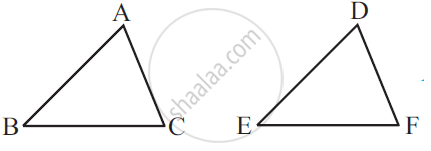Share

# Which Congruence Criterion Do You Use in the Following? Given: Ac = Df Ab = De Bc = Ef So, δAbc ≅ δDef - CBSE Class 7 - Mathematics

ConceptCriteria for Congruence of Triangles

#### Question

Which congruence criterion do you use in the following?

Given: AC = DF

AB = DE

BC = EF

So, ΔABC ≅ ΔDEF#### Solution

SSS, as the sides of ΔABC are equal to the sides of ΔDEF.

Is there an error in this question or solution?

#### APPEARS IN

NCERT Solution for Mathematics for Class 7 (2018 to Current)
Chapter 7: Congruence of Triangles
Ex. 7.20 | Q: 1 | Page no. 149
Solution Which Congruence Criterion Do You Use in the Following? Given: Ac = Df Ab = De Bc = Ef So, δAbc ≅ δDef Concept: Criteria for Congruence of Triangles.
S